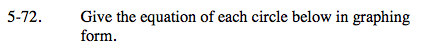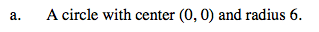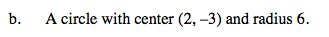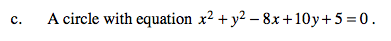### Home > A2C > Chapter 5 > Lesson 5.2.1 > Problem5-72

5-72.Remember the general equation for a circle is (xh)2 + (yk)2 = r2 where (h, k) is the center.See part (a).Complete the squares for x and y.

Rearrange the equation:
x2 − 8x + _______ + y2 + 10y + _______ = −5 + _______ + _______

(x − 4)2 + (y + 5)2 = 36

Use the eTool below to graph the equations for each part.
Click the link at right for the full version of the eTool: A2C 5-72 HW eTool